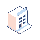• Fiyatlar

# Counting number of values in One2Many field

How exactly do i make a field that lets me show how many of my employees are in a certain department ?

Here is my code

`# -*- coding: utf-8 -*-from datetime import timedeltafrom odoo import models, fields, apiclass employee(models.Model):    _name = 'drs2.employee'    name = fields.Char(required=True)    age = fields.Integer()    height = fields.Integer()    annoying = fields.Boolean()    task_id = fields.One2many(        'drs2.task', 'employee_id', string="Tasks")    dep_id = fields.Many2one(        'drs2.dep', ondelete='cascade', string="Department")class task(models.Model):    _name = 'drs2.task'    name = fields.Char(string="Task Name", required=True)    description = fields.Text()    employee_id = fields.Many2one('drs2.employee',        ondelete='cascade', string="Employee")    dep_id = fields.Many2one(        'drs2.dep', ondelete='cascade', string="Department")    start_date = fields.Date(default=fields.Date.today)    end_date = fields.Date(default=fields.Date.today)class department(models.Model):    _name = 'drs2.dep'    name = fields.Char()    employee_id = fields.One2many(        'drs2.employee', 'dep_id', string="Employee")    count = fields.Integer(compute='count_employees')    @api.one    def count_employees(self):        return search_count(self.employee_id)`##### 2 Yanıtlar

Hello Leke Onile-ere,

Try below code :-

def count_employees(self):
for employee in self:
employee.count = self.env['drs2.employee'].search_count([('dep_id', '=', self.id)])

Hope it will works for you.

Thanks,`Try this one    @api.multi    @api.depends('employee_id')    def count_employees(self):        return len(self.employee_id)`
1 Yorum